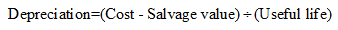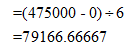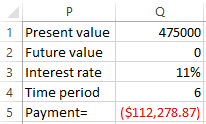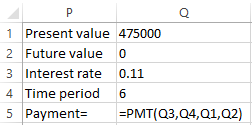# Ayden’s Toys, Inc., just purchased a \$475,000 machine to produce toy cars. The machine will be fully depreciated by the straight-line method over its 6-year economic life. Each toy sells for \$19. The variable cost per toy is \$6 and the firm incurs fixed costs of \$335,000 per year. The corporate tax rate for the company is 23 percent. The appropriate discount rate is 11 percent. What is the financial break-even point for the project?

Question
1 views

Ayden’s Toys, Inc., just purchased a \$475,000 machine to produce toy cars. The machine will be fully depreciated by the straight-line method over its 6-year economic life. Each toy sells for \$19. The variable cost per toy is \$6 and the firm incurs fixed costs of \$335,000 per year. The corporate tax rate for the company is 23 percent. The appropriate discount rate is 11 percent. What is the financial break-even point for the project?

check_circle

Step 1

With the given values, we can calculate the financial break-even point as follows:

Step 2

The formula to calculate straight line depreciation is:Substituting the values, we get the value of depreciation as:Step 3

We can calculate the payments using excel as:Hence, the payment amount is \$112,278.87

...

### Want to see the full answer?

See Solution

#### Want to see this answer and more?

Solutions are written by subject experts who are available 24/7. Questions are typically answered within 1 hour.*

See Solution
*Response times may vary by subject and question.
Tagged in